# Difference between revisions of "0708-1300/Class notes for Tuesday, November 20"

Announcements go here

## Typed Notes

The notes below are by the students and for the students. Hopefully they are useful, but they come with no guarantee of any kind.

### First Hour

Recall we are ultimately attempting to understand and prove Stokes theorem. Currently we are investigating the meaning of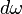$d\omega$.

Recall we had that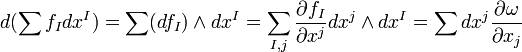$d(\sum f_I dx^I) = \sum (df_I)\wedge dx^I = \sum_{I,j}\frac{\partial f_I}{\partial x^j} dx^j\wedge dx^I = \sum dx^j\frac{\partial\omega}{\partial x_j}$

Now we want to compute$d\omega$ on the parallelepiped formed from k+1 tangent vectors. For instance let us suppose k= 2 then are interested in the parallelepiped formed from the three basis vectors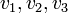$v_1, v_2, v_3$.

Feeding in the parallelepiped we get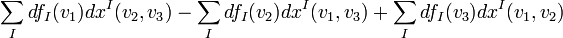$\sum_I df_I(v_1)dx^I(v_2,v_3) - \sum_I df_I(v_2)dx^I(v_1,v_3) + \sum_I df_I(v_3)dx^I(v_1,v_2)$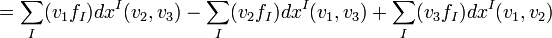$= \sum_I (v_1 f_I)dx^I(v_2,v_3) - \sum_I (v_2 f_I)dx^I(v_1,v_3) + \sum_I (v_3 f_I)dx^I(v_1,v_2)$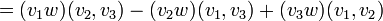$= (v_1 w)(v_2,v_3) - (v_2 w)(v_1,v_3) + (v_3 w)(v_1,v_2)$

Now,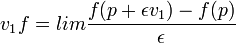$v_1 f = lim\frac{f(p+\epsilon v_1) - f(p)}{\epsilon}$, or, loosely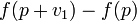$f(p+v_1) - f(p)$

So this corresponds to the difference between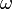$\omega$ calculated on each of the two faces parallel to the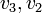$v_3,v_2$ plane.

Hence, our$d\omega$ on the parallelepiped is just the sum of parallelograms making the boundary of the parallelepiped counted with some signs.

We can see the loose idea of how the proof of stokes theorem is going to work: dividing the manifold up into little parallelepiped like this,$d\omega$ will just be the faces of the parallelepipeds and when summing over the whole manifold all of faces will cancel except those on the boundary thus just leaving the integral of$\omega$ along the boundary.

We note that this is similar to the proof of the fundamental theorem of calculus, where we take an integral and compute the value of f' at many little subintervals. But the value of f' is just the difference of f' at the boundary of each sub interval so when we add everything up everything cancels except the values of the function at the endpoint of the big interval.

Claim

d exists if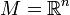$M = \mathbb{R}^n$ and is unique.

Define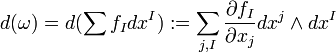$d(\omega) = d(\sum f_I dx^I) :=\sum_{j,I}\frac{\partial f_I}{\partial x_j}dx^j\wedge dx^I$

Proof

We need to check that this satisfies the properties 1) - 3) from last lecture:

1)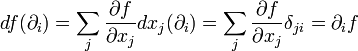$df(\partial_i) = \sum_j \frac{\partial f}{\partial x_j} dx_j(\partial_i) = \sum_j \frac{\partial f}{\partial x_j} \delta_{ji} = \partial_i f$ and so satisfies property 1)

Note

We now adopt Einstein Summation Convention which means that if in a term there is an index that is repeated, once as a subscript and once as a superscript, it is meant as implicit that we are summing over this index. This just cleans up the notation so we don't have to have sums everywhere.

2)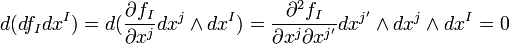$d(df_I dx^I) = d(\frac{\partial f_I}{\partial x^j} dx^j\wedge dx^I) = \frac{\partial^2 f_I}{\partial x^j \partial x^{j'}}dx^{j'}\wedge dx^j\wedge dx^I = 0$ because the mixed partial is symmetric under exchange of indices but the wedge product is antisymmetric under exchange of indices. That is, each term cancels with the one where j and j' are exchanged.

3) let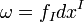$\omega=f_I dx^I$ and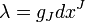$\lambda = g_J dx^J$ then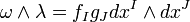$\omega\wedge\lambda = f_I g_J dx^I\wedge dx^J$

so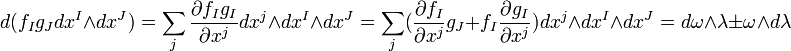$d(f_I g_J dx^I\wedge dx^J) =\sum_j \frac{\partial f_I g_I}{\partial x^j} dx^j\wedge dx^I\wedge dx^J = \sum_j (\frac{\partial f_I}{\partial x^j}g_J + f_I\frac{\partial g_I}{\partial x^j})dx^j\wedge dx^I\wedge dx^J = d\omega\wedge\lambda \pm\omega\wedge d\lambda$

Via assignment 3 this is unique.

Q.E.D.

Now, we can extend this definition on manifolds by using coordinate charts.

Claim

Properties 1-3 imply that on any M, d is local. That is, if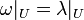$\omega|_U = \lambda|_U$ then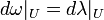$d\omega|_U = d\lambda|_U$

Proof: Exercise.

Definition

For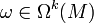$\omega\in\Omega^k(M)$ the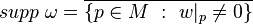$supp\ \omega = \overline{\{p\in M\ :\ w|_p\neq0\}}$

Then$\omega$ has compact support if the supp$\omega$ is compact.

Define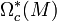$\Omega^*_c(M)$ := the compactly supported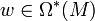$w\in\Omega^*(M)$

Definition

For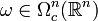$\omega\in\Omega^n_c(\mathbb{R}^n)$,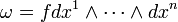$\omega = fdx^1\wedge\cdots\wedge dx^n$ we define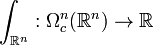$\int_{\mathbb{R}^n}: \Omega^n_c(\mathbb{R}^n)\rightarrow\mathbb{R}$ by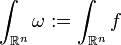$\int_{\mathbb{R}^n}\omega := \int_{\mathbb{R}^n}f$

I.e.,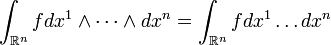$\int_{\mathbb{R}^n}fdx^1\wedge\cdots\wedge dx^n = \int_{\mathbb{R}^n}fdx^1\ldots dx^n$

### Second Hour

In general if we have a diffeomorphism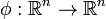$\phi:\mathbb{R}^n \rightarrow\mathbb{R}^n$ then the normal integral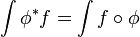$\int \phi^*f = \int f\circ\phi$ is not equal to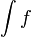$\int f$

However we claim that this IS true for differential forms. I.e.,

Claim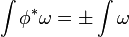$\int \phi^*\omega = \pm\int \omega$ as forms

This is very important because it essentially means we can integrate in whatever charts we like and get the same thing.

Proof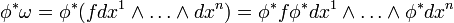$\phi^*\omega = \phi^*(fdx^1\wedge\ldots\wedge dx^n) = \phi^* f\phi^* dx^1\wedge\ldots\wedge \phi^* dx^n$

Now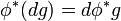$\phi^* (dg) = d\phi^* g$ by chain rule and so we extend to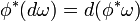$\phi^*(d\omega) = d(\phi^*\omega)$

Hence,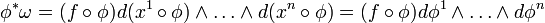$\phi^*\omega = (f\circ\phi) d(x^1\circ\phi)\wedge\ldots\wedge d(x^n\circ\phi) = (f\circ\phi) d\phi^1\wedge\ldots\wedge d\phi^n$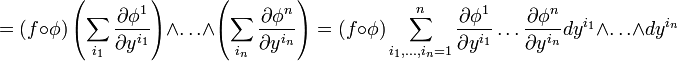$= (f\circ\phi)\left(\sum_{i_1} \frac{\partial\phi^1}{\partial y^{i_1}}\right)\wedge\ldots\wedge\left(\sum_{i_n}\frac{\partial\phi^n}{\partial y^{i_n}}\right) = (f\circ\phi)\sum_{i_1,\ldots,i_n =1}^n \frac{\partial\phi^1}{\partial y^{i_1}}\ldots\frac{\partial\phi^n}{\partial y^{i_n}}dy^{i_1}\wedge\ldots\wedge dy^{i_n}$

but the wedge product is zero unless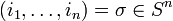$(i_1,\ldots,i_n)=\sigma\in S^n$ and in that case yields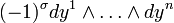$(-1)^{\sigma} dy^1\wedge\ldots\wedge dy^n$

Hence we get,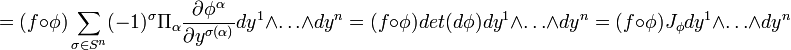$=(f\circ\phi)\sum_{\sigma\in S^n}(-1)^{\sigma}\Pi_{\alpha} \frac{\partial\phi^{\alpha}}{\partial y^{\sigma(\alpha)}}dy^1\wedge\ldots\wedge dy^n = (f\circ\phi) det (d\phi)dy^1\wedge\ldots\wedge dy^n = (f\circ\phi)J_{\phi}dy^1\wedge\ldots\wedge dy^n$

where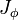$J_{\phi}$ is the determinant of the Jacobian matrix.

Hence,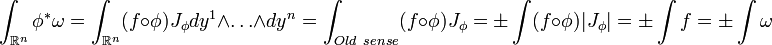$\int_{\mathbb{R}^n} \phi^*\omega = \int_{\mathbb{R}^n}(f\circ\phi)J_{\phi}dy^1\wedge\ldots\wedge dy^n = \int_{Old\ sense} (f\circ\phi)J_{\phi} =\pm \int (f\circ\phi)|J_{\phi}| = \pm\int f = \pm\int \omega$

Q.E.D

If we restrict our attention to just the orientation preserving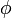$\phi$'s so that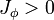$J_{\phi}>0$ then we will always get the + in the end.

Definition

An orientation of M is an assignment of the charts to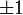$\pm 1$,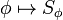$\phi\mapsto S_{\phi}$

Then if the domains on$\phi$ and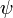$\psi$ overlap then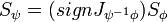$S_{\psi} = (sign J_{\psi^{-1}\phi})S_{\phi}$

By definition, M is orientable if we can find an orientation.

Examples

1)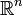$\mathbb{R}^n$. We declare the identity positive and then all other designations follow from this.

2) The finite cylinder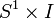$S^1\times I$

We can put two charts on the cylinder by considering two rectangles which overlap by a little bit that cover the whole cylinder. If we denote one of these as positive, the overlap makes the other positive. We compare any other chart to these two.

3) Consider the mobius strip and the same attempted charts as for the cylinder. If we label one section positive, the other section must be positive due to the overlap on one side, but must be negative due to the overlap on the other side. Thus the mobius strip is not orientable.

Definition

An orientation of a vector space V is an equivalence class of ordered bases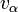$v_{\alpha}$ of V, where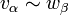$v_{\alpha}\sim w_{\beta}$ if the determinant of the transition matrix between these two bases is positive.

Definition

An orientation of M is a continuous choice of orientations for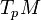$T_p M$ for any p. We haven't technically defined what it means to be continuous in this sense but the meaning is clear.

Definition

let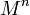$M^n$ be an oriented manifold and let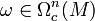$\omega\in\Omega^n_c(M)$. Let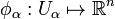$\phi_{\alpha}:U_{\alpha}\mapsto\mathbb{R}^n$ be a collection of positive charts that cover M. Let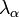$\lambda_{\alpha}$ be a partition of unity subordinate to this cover then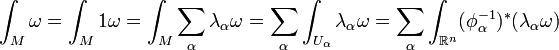$\int_M\omega = \int_M 1\omega = \int_M \sum_{\alpha}\lambda_{\alpha}\omega = \sum_{\alpha}\int_{U_{\alpha}}\lambda_{\alpha}\omega = \sum_{\alpha}\int_{\mathbb{R}^n} (\phi^{-1}_{\alpha})^*(\lambda_{\alpha}\omega)$

Note all the intermediate steps were merely properties we would LIKE the integral to have, the actual definition is the equality of the left most and right most expressions.

Theorem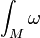$\int_M\omega$ is independent of the choices.